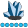plf515
Lapis Lazuli | Level 10

## How can I graph complex interactions from regression?

Hello

I have modelled regressions that include various quadratics and intereactions.  I have saved the predicted values so, for each model I have

V1    V2    Pred

1        2       5

1.1     1.2    4.30

etc for several hundred lines

I'd like a graph that has V1 on the x axis, Pred on the y axis and lines for different quantiles of V2.

I know I've done this before.... but I can't remember how.

TIA

Peter

1 ACCEPTED SOLUTION

Accepted Solutions

## Re: How can I graph complex interactions from regression?

If V2 is a class variable, you can use a SERIES statement with GROUP= option:

SERIES x=v1 y=pred / group=v2;

However, if V2 is continuous, this won't work so well.  Instead, use the EFFECTPLOT statement to get what you want.  It sounds like you might want to use the SLICEFIT option with the SLICEBY= suboption, as shown in the second example that I linked to.

If your regression procedure does not support the EFFECTPLOT statement directly, you can STORE the  model to an item store and use PROC PLM, which does support the EFFECTPLOT statement.

## Re: How can I graph complex interactions from regression?

If V2 is a class variable, you can use a SERIES statement with GROUP= option:

SERIES x=v1 y=pred / group=v2;

However, if V2 is continuous, this won't work so well.  Instead, use the EFFECTPLOT statement to get what you want.  It sounds like you might want to use the SLICEFIT option with the SLICEBY= suboption, as shown in the second example that I linked to.

If your regression procedure does not support the EFFECTPLOT statement directly, you can STORE the  model to an item store and use PROC PLM, which does support the EFFECTPLOT statement.

Discussion stats